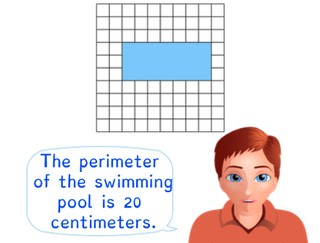Determining simple perimeter using squares

# Determining simple perimeter using squares

The students learn to determine simple perimeter using squares.8,000 schools use Gynzy92,000 teachers use Gynzy1,600,000 students use Gynzy

## General

The students learn how they can determine the perimeter of a shape using squares.

## Standards

CCSS.Math.Content.3.MD.D.8

## Learning objective

Students will be able to calculate a perimeter using squares.

## Introduction

A number of pigs are shown on the interactive whiteboard. The students must state how many pigs there are and how they counted them in steps. Repeat this for the jars and the erasers.

## Instruction

Ask if the students can explain what perimeter is. Explain that the perimeter shows how long something is if you measure around it. It is the edge of the shape. You can determine how large the perimeter is in multiple ways. For a simple shape, such as a square or a rectangle, you can count the squares around the shape one by one. You can also use the multiplication tables. So you do the length × 2 and the width × 2. You then add the outcomes together. Show a number of shapes for which the students must determine the perimeter. Ask how they calculated this. Explain that you can't always count the squares. If a shape is not a square or a rectangle, then you have to count the sides. Next you add the sides together. Show several shapes and ask what the perimeter is. Erase the cover to show what the answer is. After this you show other shapes for which the students must give the perimeter.

To check whether the students know how to determine perimeter, you can ask the following question:
- What methods can you use to determine perimeter?

## Quiz

The students test their understanding of determining simple perimeter using squares through ten exercises. In each of the exercises, they must determine the perimeter of the rectangle or square given. Some of the exercises have possible answers provided, and for others the students must find the answer on their own.

## Closing

You discuss with the students again that it is important to be able to determine perimeter with squares, because then you can more quickly calculate what the perimeter is. Have the students practice in pairs with determining the perimeter using squares. Hand out graphing paper and have the students draw a shape. This cannot be a square or rectangle, it must be a more complicated shape. The pairs trade their drawing and the other must give the perimeter of the shape.Je bespreekt met de leerlingen nog eens dat het handig is om de omtrek te kunnen berekenen via hokjes, omdat je zo snel kunt uitrekenen wat de omtrek is. Laat de leerlingen in tweetallen oefenen met het bepalen van de omtrek via hokjes. Deel hokjespapier uit en laat de leerlingen een figuur tekenen. Dit mag een rechthoek of vierkant zijn, maar een een iets ingewikkelder figuur. De tweetallen wisselen hun tekening uit en ze moeten aangeven wat de getekende omtrek is

## Teaching tips

When students have difficulty calculating perimeter using squares, then have them write a number in each of the squares around the shape, so that they don't count a square twice or miss one.

## Instruction materials

Graphing paper

### The online teaching platform for interactive whiteboards and displays in schools

• Save time building lessons

• Manage the classroom more efficiently

• Increase student engagement